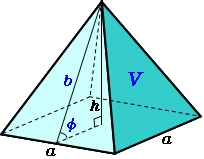Calculate the height and surface of a regular quadrilateral pyramid with a base edge a = 8 cm and a wall height w = 10 cm

h =  9.1652 cm
S =  224 cm2

### Step-by-step explanation:Did you find an error or inaccuracy? Feel free to write us. Thank you!

Tips for related online calculators
The Pythagorean theorem is the base for the right triangle calculator.

#### You need to know the following knowledge to solve this word math problem:

We encourage you to watch this tutorial video on this math problem: